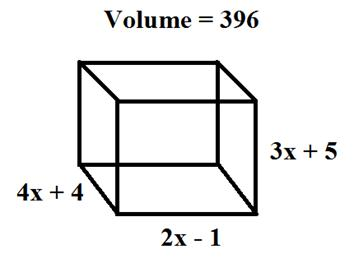### Sample Problem

The dimensions of a water tank are: height = 3x + 5 inches, length = 2x – 1 inches, and width = 4x + 4 inches. If the volume of the water tank is 396 cubic inches, what is the value of x?x = inches

#### Solution

Volume=hlw

396 = (3x + 5)(4x + 4)(2x-1)

24x3+52x2+8x-416=0

4(x-2)(6x2+25x+52)=0

x = 2